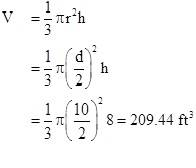# Volume of a Cylinder

• jim1174

## Homework Statement

How would you calculate the volume of a cylindrical tank that is on its side (called a horizontal tank)? Calculate the volume if that tank that is 10 ft. in diameter and 18 ft. long. I already know the answer i just need help understanding how they got the answer

## The Attempt at a Solution

[/B]## Homework Statement

How would you calculate the volume of a cylindrical tank that is on its side (called a horizontal tank)? Calculate the volume if that tank that is 10 ft. in diameter and 18 ft. long. I already know the answer i just need help understanding how they got the answer

## The Attempt at a Solution

[/B]The radius of the tank is equal to half the diameter: d = 10 feet → r = 10/2 = 5 feet.

Since the tank is on its side, its length will be equal to the height of a similar cylindrical tank standing on its end, thus h = length = 10 feet.

Plug numbers into formula, turn crank, out pops volume.

Only, in this case, the factor of 1/3 doesn't belong in the formula for the volume of a cylindrical tank. Are you sure that the tank wasn't in the shape of a cone or conical?

is this right
volume= pi x (5ft)2 x18
3.14 x 25ft2 x18 ft
35325ft

is this right
volume= pi x (5ft)2 x18
3.14 x 25ft2 x18 ft
35325ft
No. You've multiplied 3.14 by 252 by 18, instead of multiplying 3.14 by 25 by 18.

is this right
volume= pi x (5ft)2 x18
3.14 x 25ft2 x18 ft
35325ft
Also, the units are usually omitted. If you put them in, be consistent. You have units for the radius but left them out for the length of the tank. It should be understood by a reader that the units on the left and right sides are the same; namely, ft3.

so the correct answer is 3.14 X 25 X18 = 1413ft

so the correct answer is 3.14 X 25 X18 = 1413ft
No, for two reasons.
1. For a volume with measurements in feet, the volume units are cubic feet.
2. The correct answer is closer to 1414 cu. ft. Because you used such a crude approximation for ##\pi##, it caused the precision of your calculated volume to be off in the units place.

this is what is written on my home work sheet
For a cylindrical tank, the formula to determine volume is:

Volume = π r2 h where:
π = pi, a mathematical constant that equals approximately 3.14

h = height of the tank

Example: A cylindrical tank is 10 ft. in diameter and 20 ft. tall; what is its volume?

r = 1/2d = ½ x 10 ft = 5 ft.

h = 20 ft.

Volume = π x (5 ft)2 x 20 ft

= 3.14 x 25 ft.2 x 20 ft

= 1570 ft3

this is what is written on my home work sheet
For a cylindrical tank, the formula to determine volume is:

Volume = π r2 h where:
Please do something to indicate that a number is an exponent. The simplest thing to do is to use the ^ symbol, as in πr^2 * h.
jim1174 said:
π = pi, a mathematical constant that equals approximately 3.14

h = height of the tank

Example: A cylindrical tank is 10 ft. in diameter and 20 ft. tall; what is its volume?

r = 1/2d = ½ x 10 ft = 5 ft.

h = 20 ft.

Volume = π x (5 ft)2 x 20 ft

= 3.14 x 25 ft.2 x 20 ft

= 1570 ft3
Using 3.14 for ##\pi##, the above is the answer you get, but you should be using a better approximation. Most calculators have ##\pi## built in with about 10 decimal place accuracy. Using a more accurate value, the answer is closer to 1571 ft3

i think i have figured out the answer to my original question
V = ( pi / 4 ) ( D )^2 ( L )

V = ( pi / 4 ) ( 10 ft )^2 ( 18 ft )

V = 450 pi ft^3 = 1413 cu ft

i think i have figured out the answer to my original question
V = ( pi / 4 ) ( D )^2 ( L )

V = ( pi / 4 ) ( 10 ft )^2 ( 18 ft )

V = 450 pi ft^3 = 1413 cu ft
If you round to the nearest cubic foot, it's 1414 cu. ft. Apparently you're still using 3.14 for ##\pi##, which is a very crude approximation.